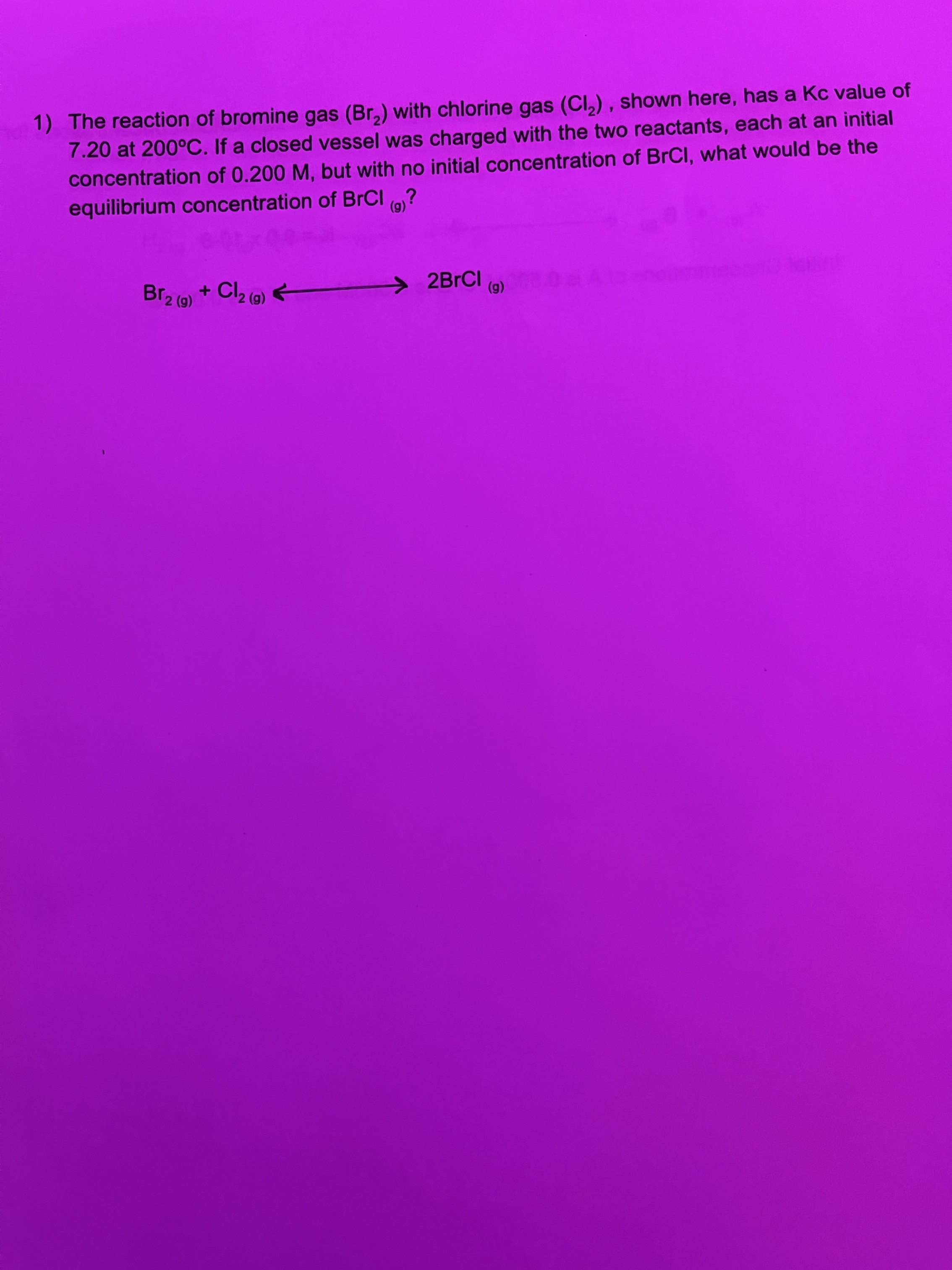# Help Zone

### Student QuestionI don’t understand this. Is someone able to show me all the work ? I’m so confused with ice and quadratic equations

Chemistry## Explanations (1)

•Explanation from AlloprofExplanation from Alloprof

This Explanation was submitted by a member of the Alloprof team.

Options
Team Alloprof • 1mo. edited April 13

Questions like this often involve using algebra to replace unknown concentration values. But first, let's write the equilibrium constant equation:

$$K_c = \frac{[BrCl_2]^2}{[Br_2][Cl_2]}$$

$$7.2 = \frac{[BrCl_2]^2}{[Br_2][Cl_2]}$$

Its is easy to realize that we are missing three variables, and thus, cannot simply solve the equation for the concentration of BrCl2. Therefore, we must use variables.

First, let's establish that the concentration of Br2 and Cl2 at equilibrium state is 0.2-x. Indeed, both reactants start at a concentration of 0.2 and lose x moles during the reaction to form BrCl.

Because there were 0 moles of BrCl to start of with, we can establish that the number of moles of BrCl at equilibrium state will be 2x (because for every x moles of Br and Cl lost, there are 2x moles of BrCl that are produced).

We can now rewrite the equilibrium constant reaction:

$$7.2 = \frac{[BrCl_2]^2}{[Br_2][Cl_2]} = \frac{(2x)^2}{(0.2-x)(0.2-x)}$$

Now, we can isolate x to find the final concentration of BrCl:

$$7.2 = \frac{4x^2}{0.04-0.4x+x^2}$$

$$7.2(0.04-0.4x+x^2) = 4x^2$$

$$7.2x^2 - 2.88x + 0.288 = 4x^2$$

$$3x^2 - 2.88x + 0.288 = 0$$

At this point, we have to apply the quadratic formula. In this case, a = 3, b = - 2.88 and c = 0. 288

Remember that the equation for the quadratic equation is:

$$x_{1,2}=\dfrac{-b\pm\sqrt{b^2-4ac}}{2a}$$

I will let you solve the quadratic equation. To choose between x values, remember that x cannot be bigger than 0.4 (because there were only 0.2 moles of reactants to start with).

This webpage from the Alloprof website is about the equilibrium constant:

Don't hesitate to ask for more help!